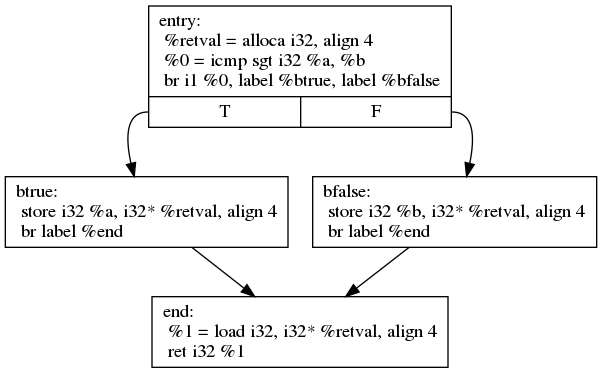# Simple “if-then-else” Branching¶

First let’s take a look at a very simple function, that computes the maximum of two integers. This is implemented using a single if control statement.

```int max(int a, int b) {
if (a > b) {
return a;
} else {
return b;
}
}
```

Remember that in LLVM IR control-flow is implemented by jumping between basic blocks, which contain instruction sequences that do not change control flow. Each basic block ends with an instruction that changes the control flow. The most common branching instruction is `br` . `br` can be used conditionally, then it implements a simple if-then-else, taking a boolean condition flag and two basic block labels as parameter.

```br i1 %cond, label %iftrue, label %iffalse
```

`br` can also be used to unconditionally jump to a certain destination:

```br label %dest
```
```define i32 @max(i32 %a, i32 %b) {
entry:
%retval = alloca i32, align 4
%0 = icmp sgt i32 %a, %b
br i1 %0, label %btrue, label %bfalse

btrue:                                      ; preds = %2
store i32 %a, i32* %retval, align 4
br label %end

bfalse:                                     ; preds = %2
store i32 %b, i32* %retval, align 4
br label %end

end:                                     ; preds = %btrue, %bfalse
%1 = load i32, i32* %retval, align 4
ret i32 %1
}
```

In the example above, there are 4 basic blocks. The first one is the function entry block. There space is allocated on the stack with `alloca` , which acts as a temporary storage for the bigger value. Then the two parameter `%a` and `%b` are compared using the `icmp` instruction . The result is a boolean (`i1`) flag, which is then used as condition for the `br` instruction. Then depending on the taken branch, either `%a` or `%b` is stored into the temporary `%retval` variable. Each of the branches then end with a unconditional branch to the last basic block `%end`. There the value from `%retval` is loaded and returned.

You can get a graphical representation of the control-flow in the form of a control-flow graph (CFG). This can be generated by using `opt -dot-cfg input.ll`.Control-Flow Graph of the max function

LLVM IR is a rather rich intermediate code format. So when compiling the above snippet with higher optimization levels, LLVM will optimize the code to use the `select` instruction  instead of generating branches. The `select` instruction simply chooses between two values, based on a boolean condition. This shortens the code significantly.

```define i32 @max(i32 %a, i32 %b) {
%1 = icmp sgt i32 %a, %b
%2 = select i1 %1, i32 %a, i32 %b
ret i32 %2
}
```